# RD Sharma Solutions For Class 12 Maths Exercise 17.2 Chapter 17 Increasing and Decreasing Functions

RD Sharma Solutions for Class 12 Maths Exercise 17.2 Chapter 17 Increasing and Decreasing Functions is available here. This exercise explains the monotonicity of a given function. RD Sharma Solutions are framed by a team of experts who have extensive knowledge about the respective subjects. The PDF of solutions of exercise 17.2 primarily helps students from the exam point of view and by practising regularly, students can obtain worthy results in the Class 12 exams. The PDF of RD Sharma Solutions for Class 12 Maths Chapter 17 Exercise 17.2 is available here. Some of the important topics of this exercise are listed below.

• Necessary and sufficient conditions for monotonicity
• Finding the intervals in which a function is increasing or decreasing
• Proving the monotonicity of a function on a given interval
• Finding the interval in which a function is increasing or decreasing

## RD Sharma Solutions For Class 12 Maths Chapter 17 Increasing and Decreasing Functions Exercise 17.2: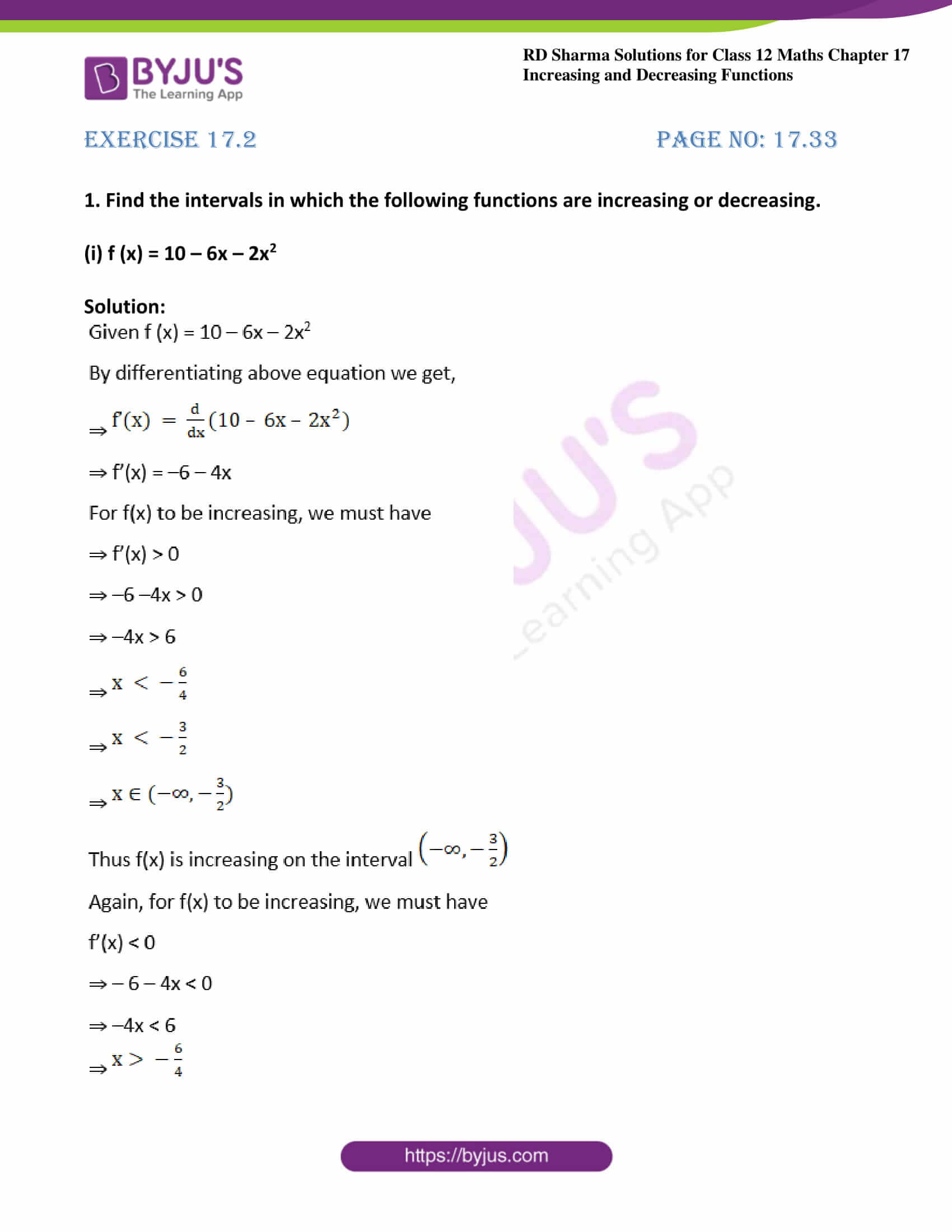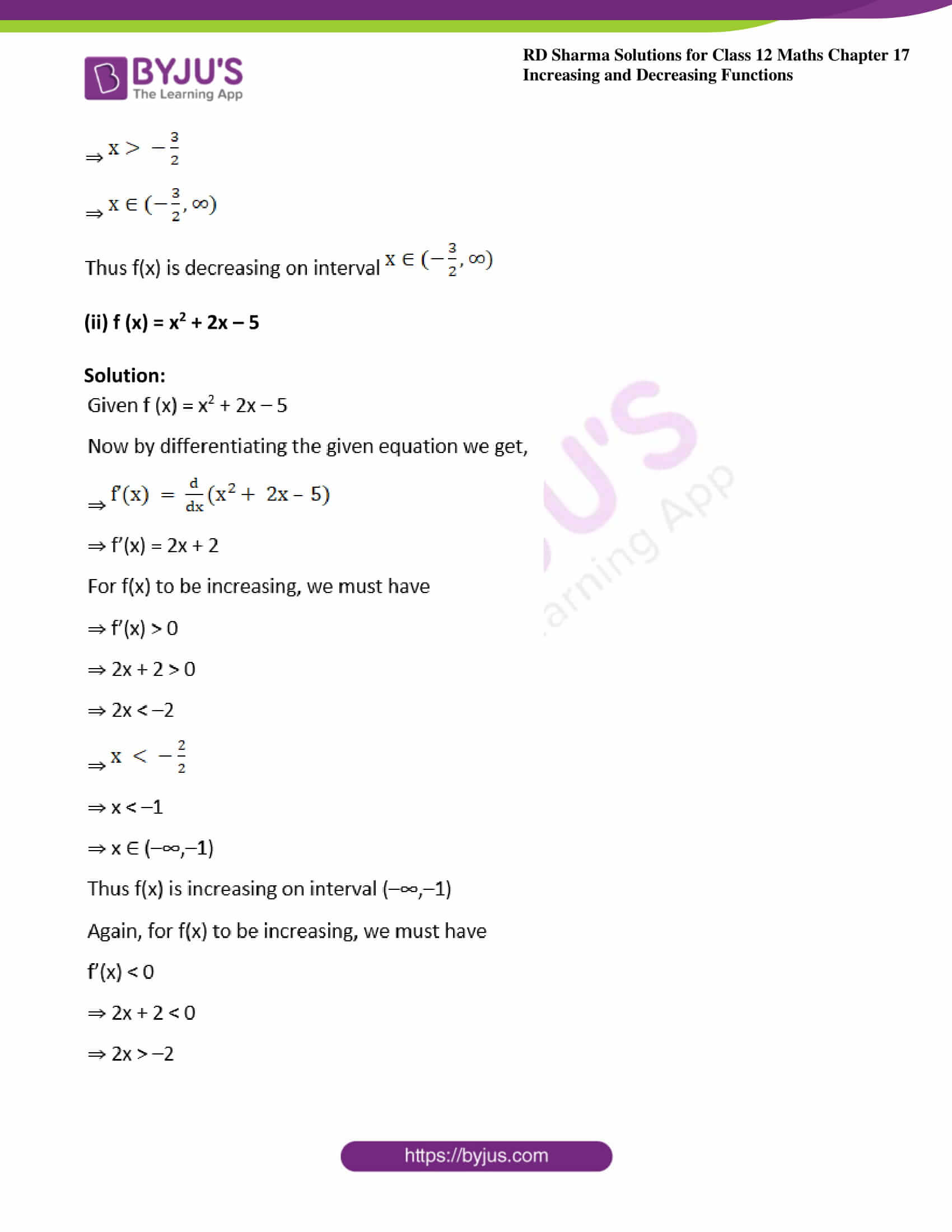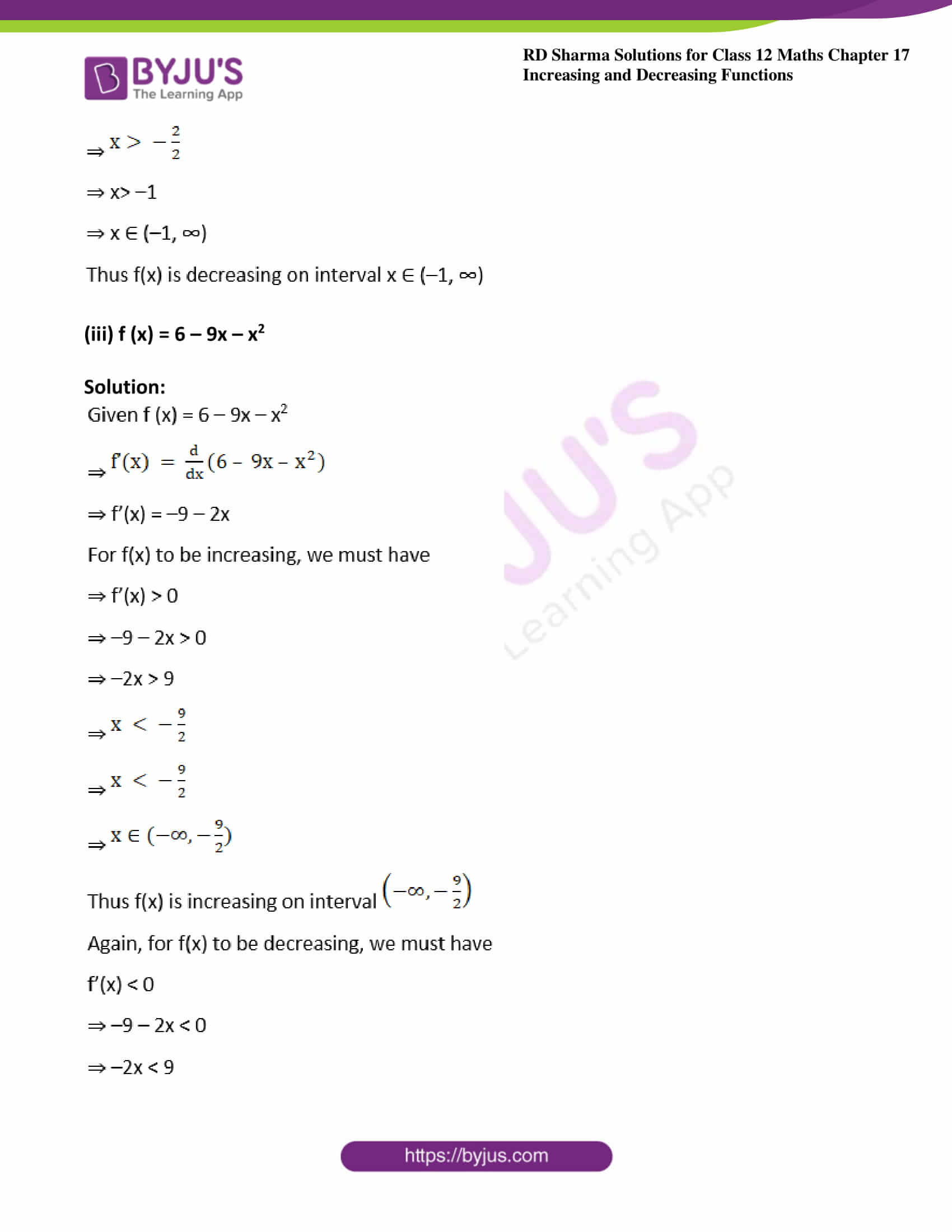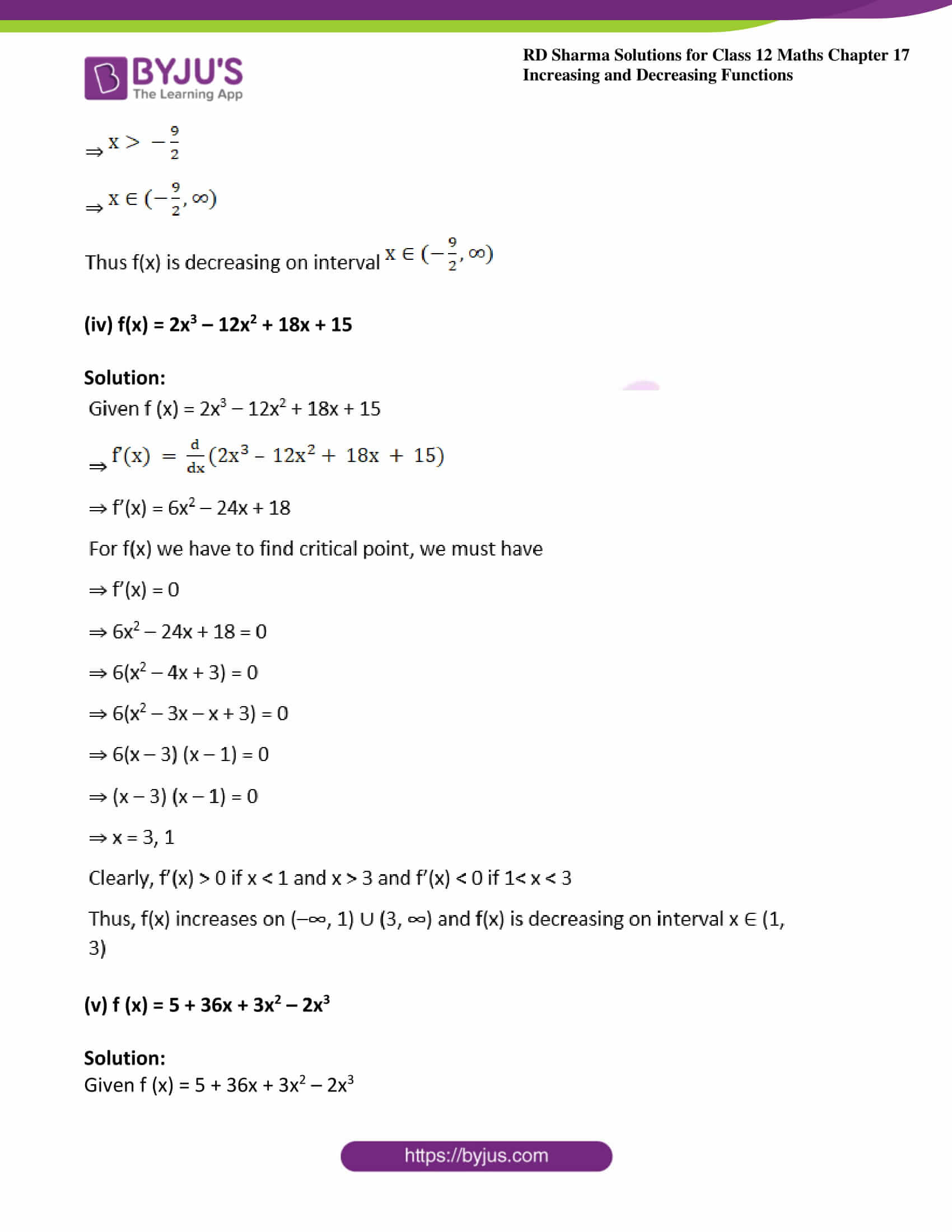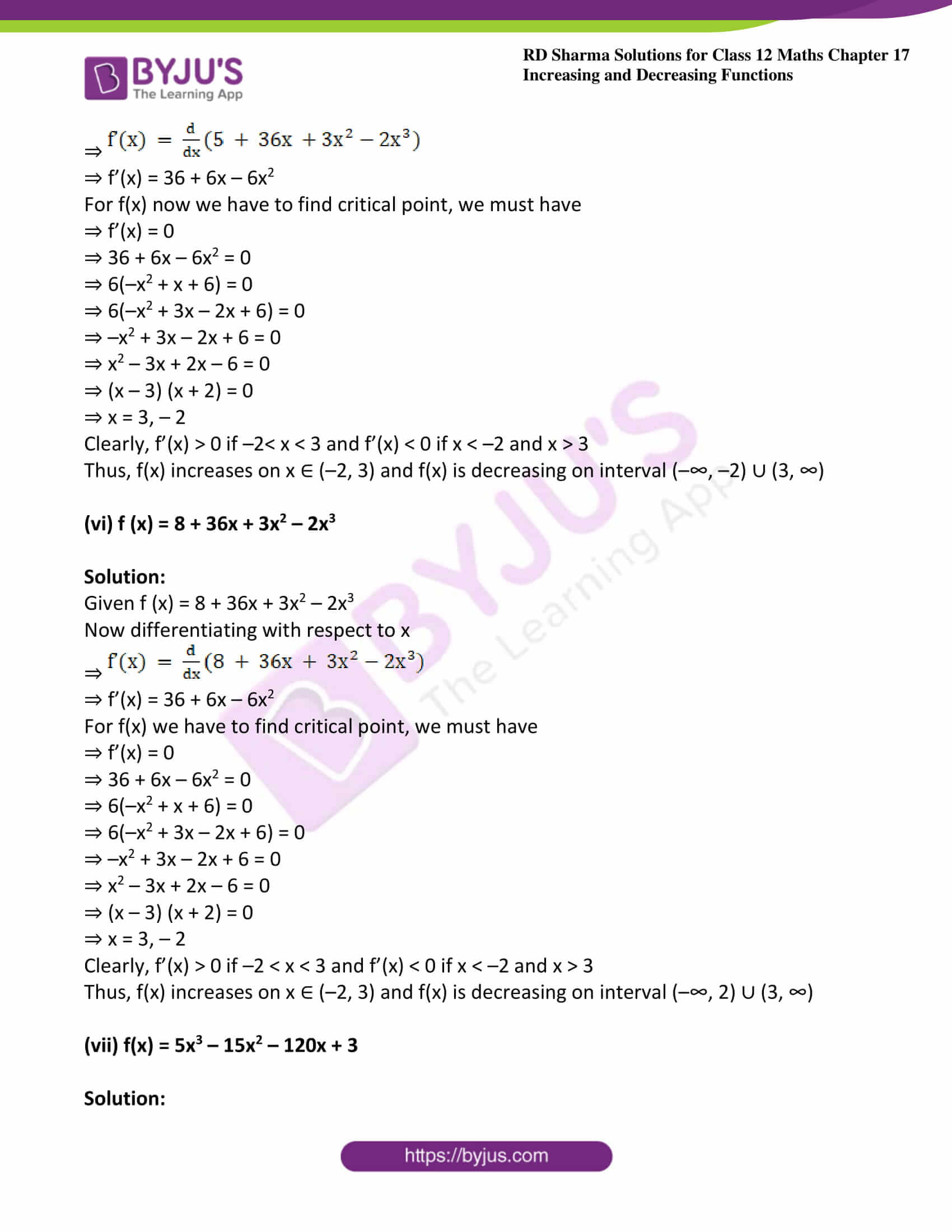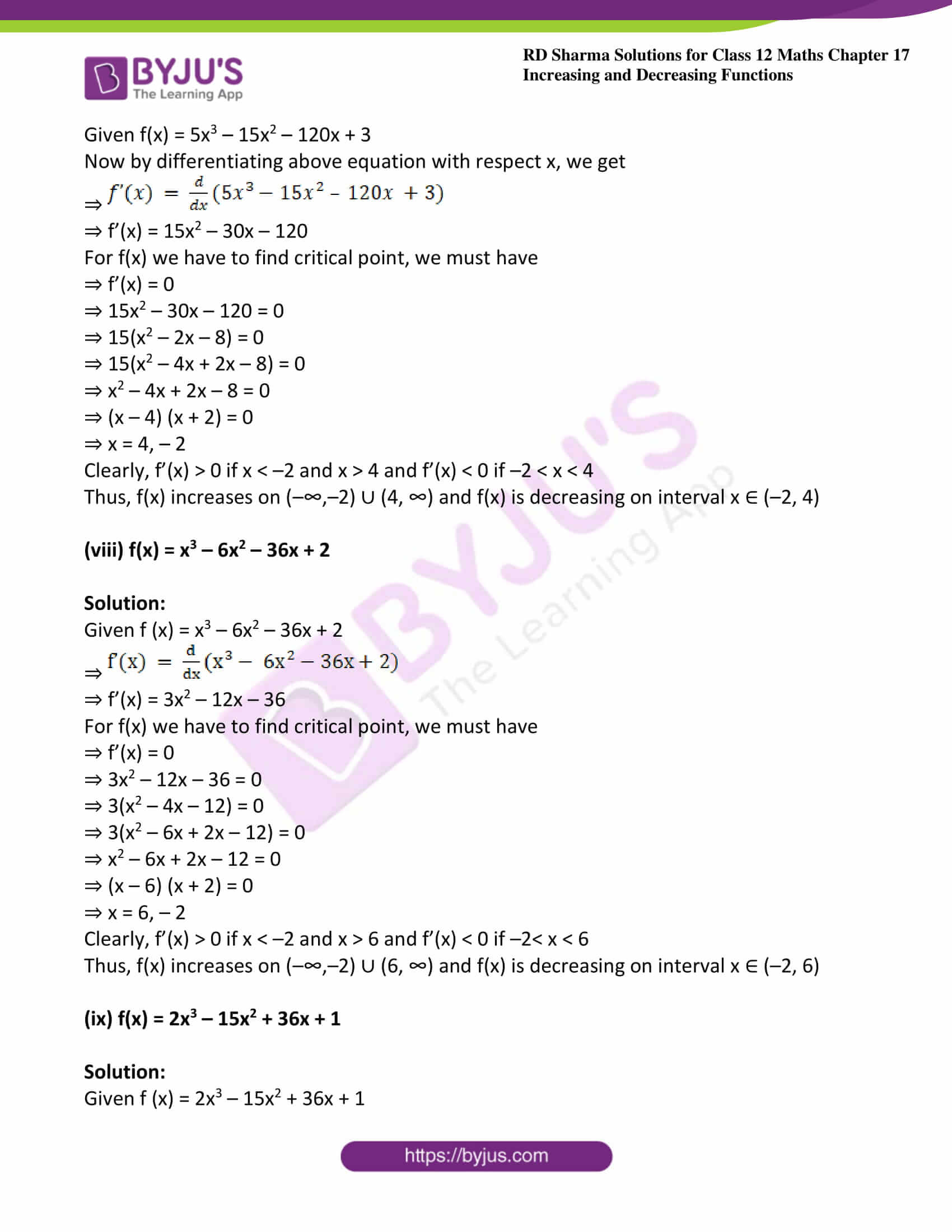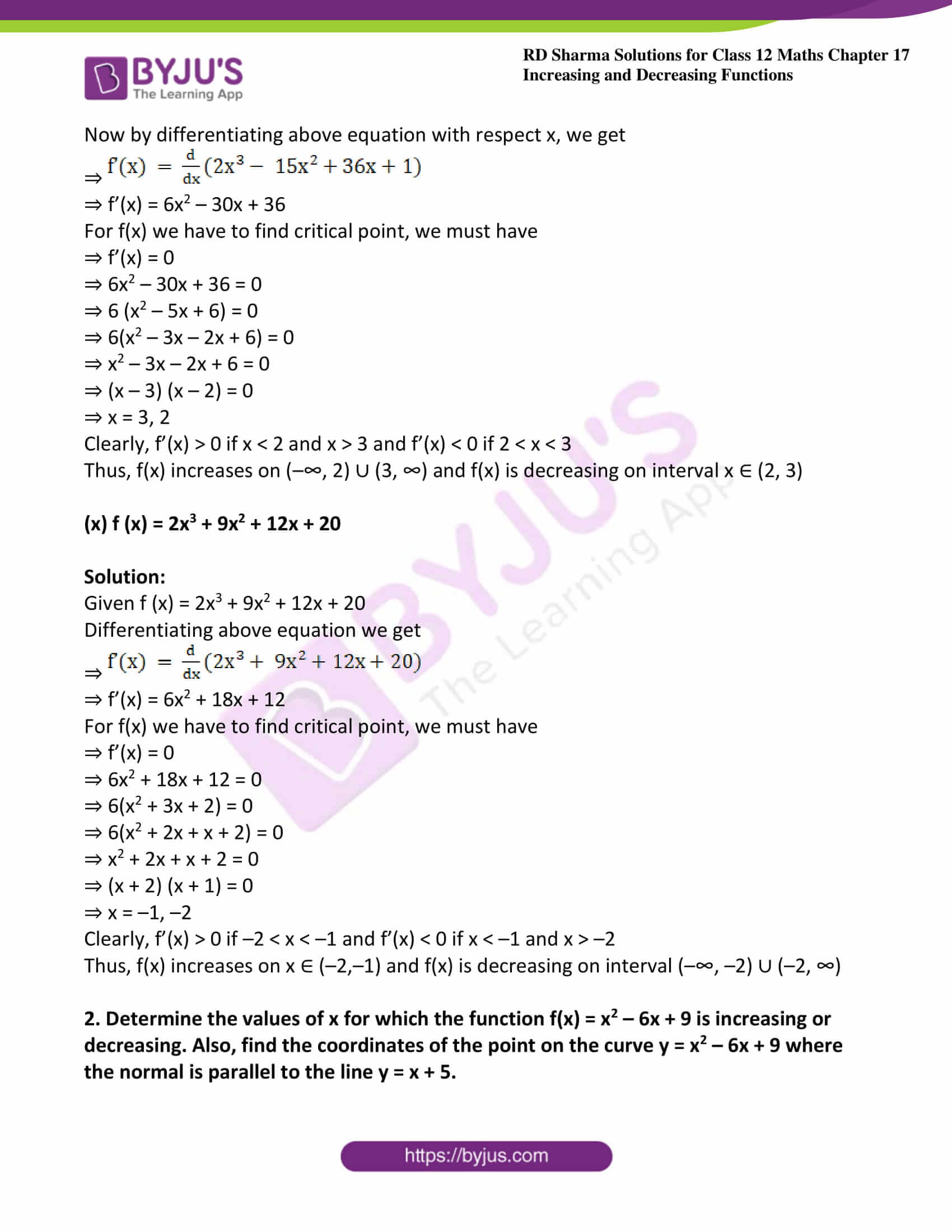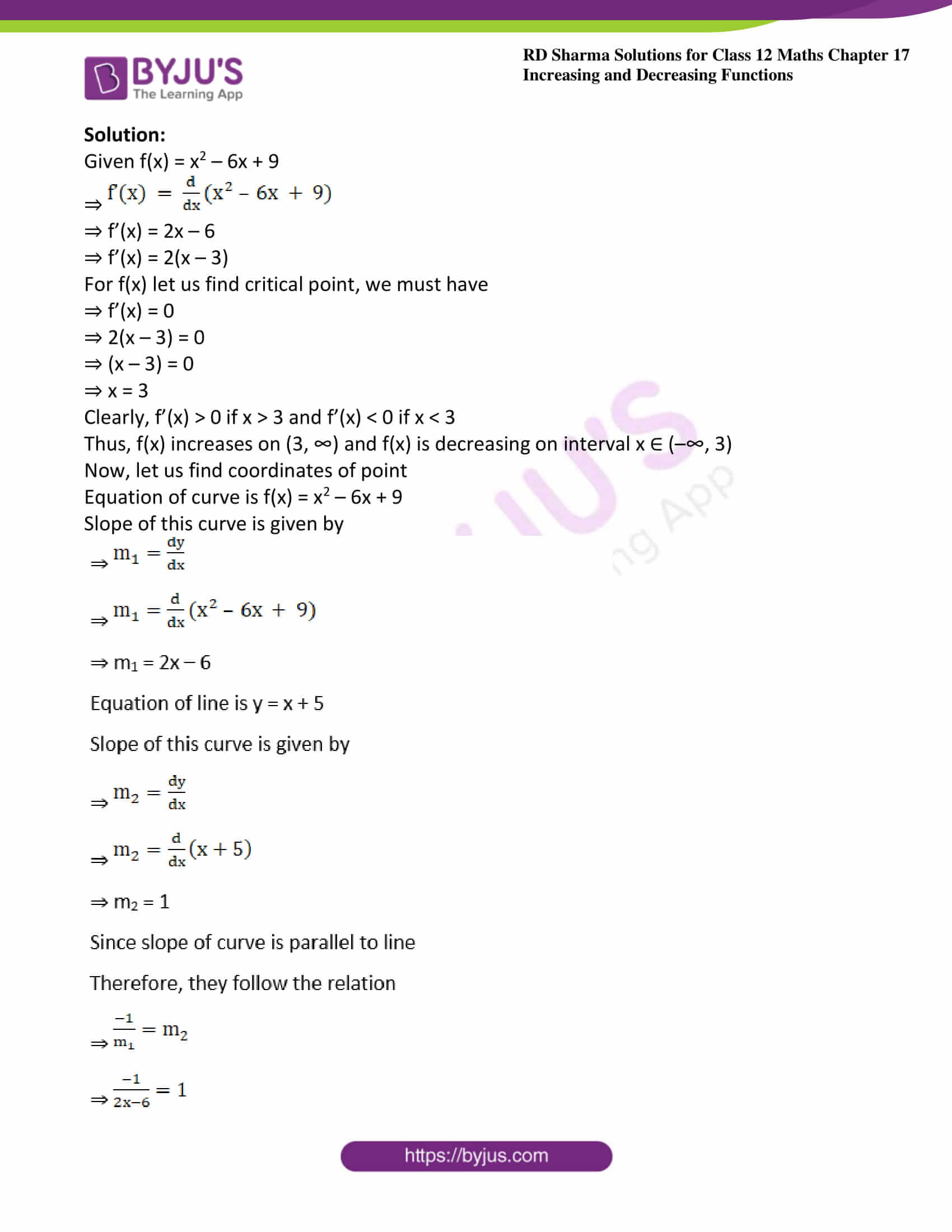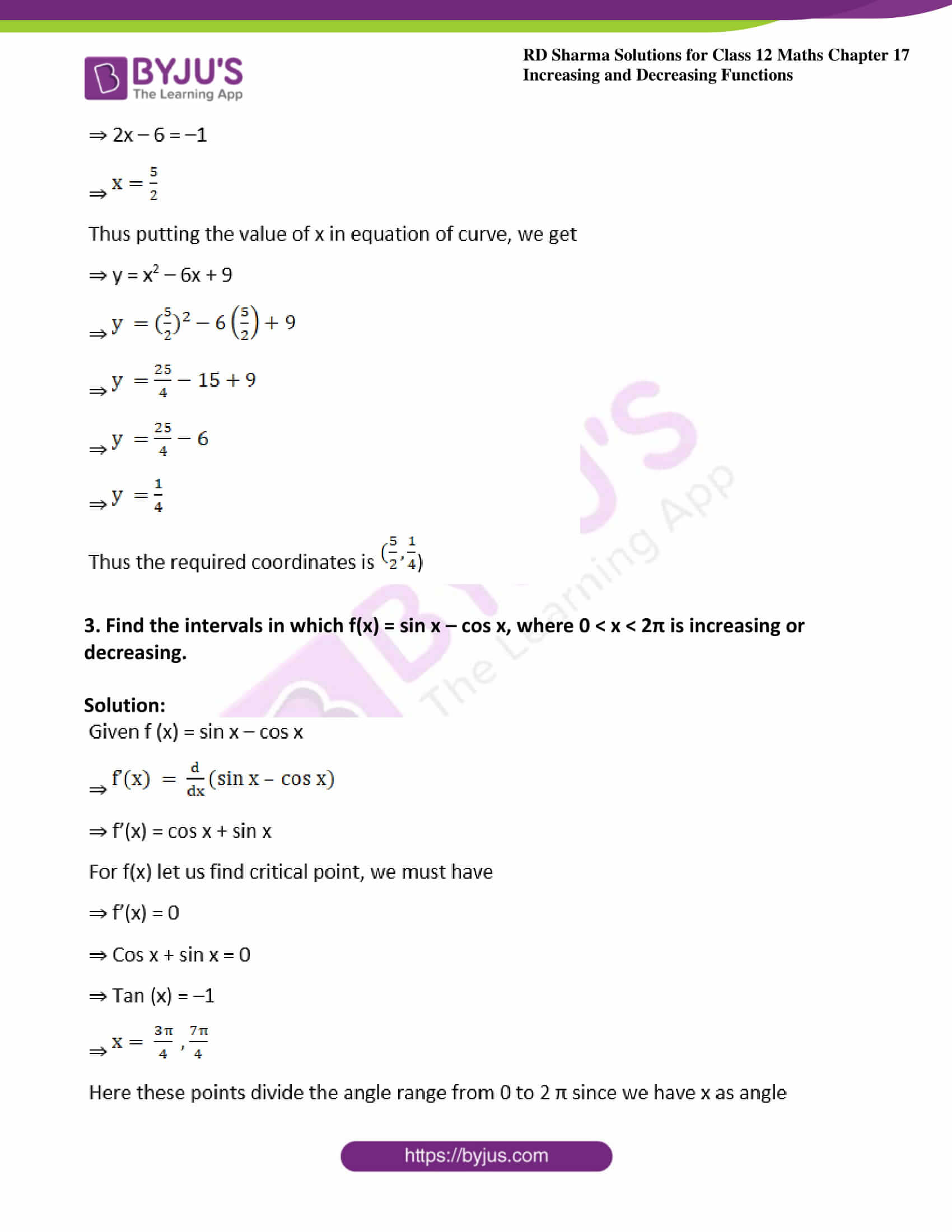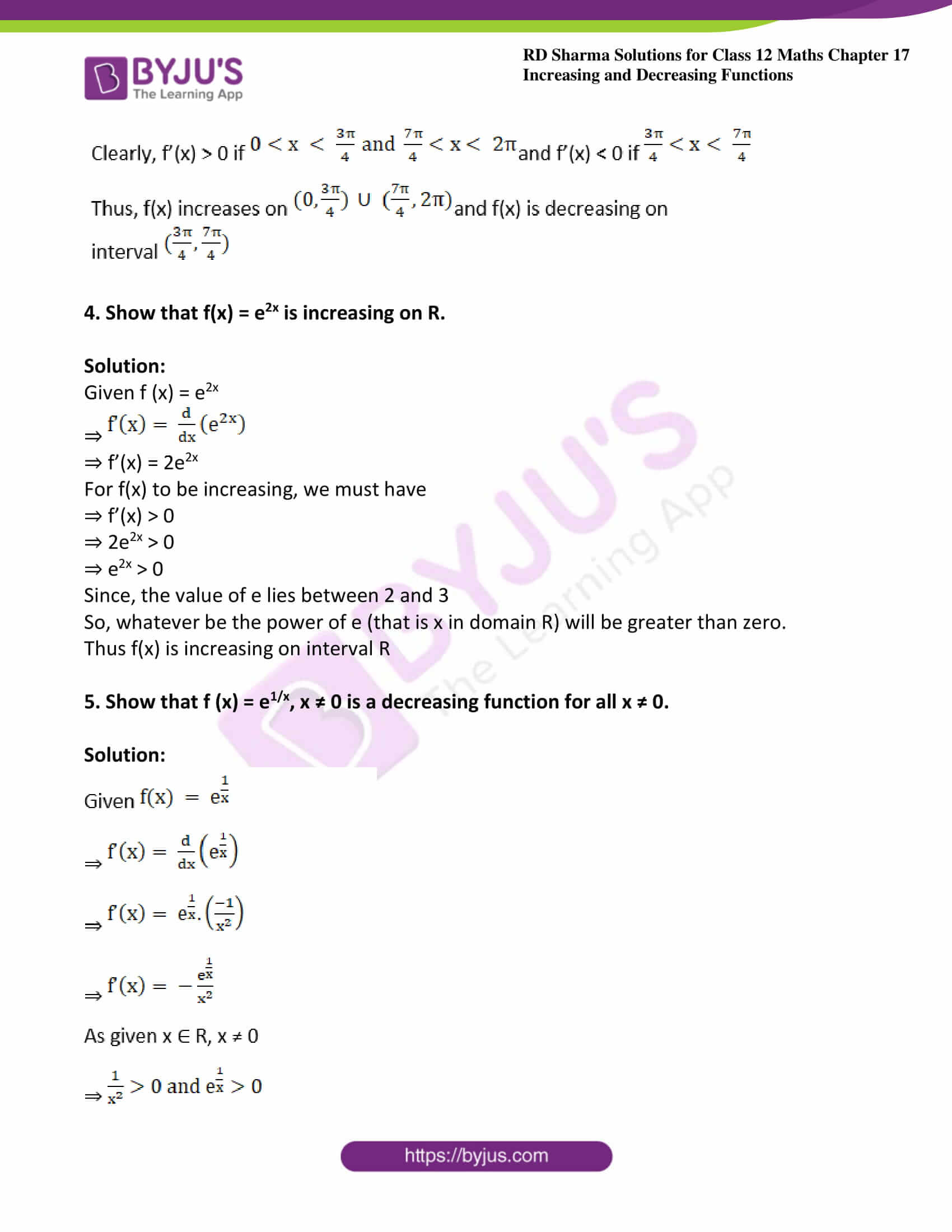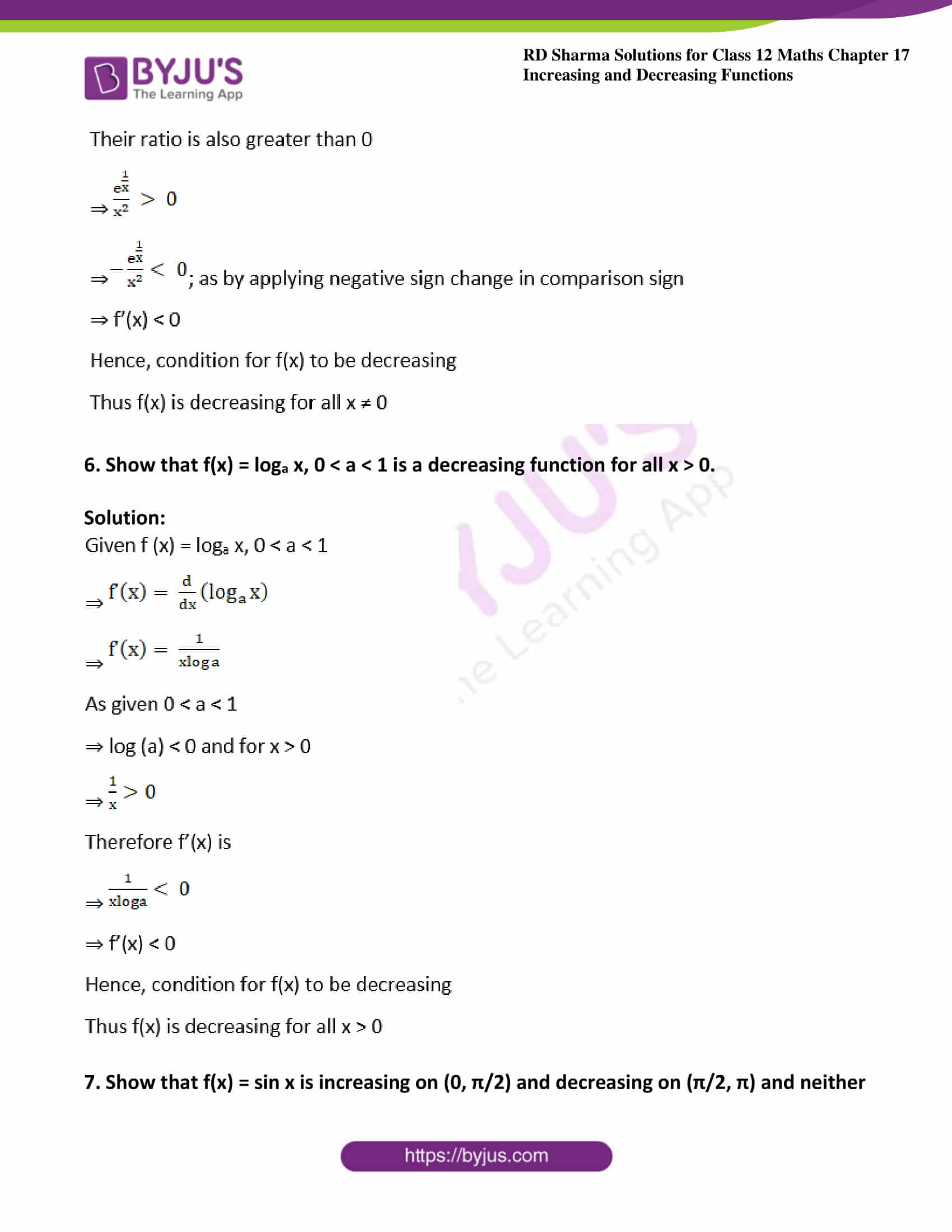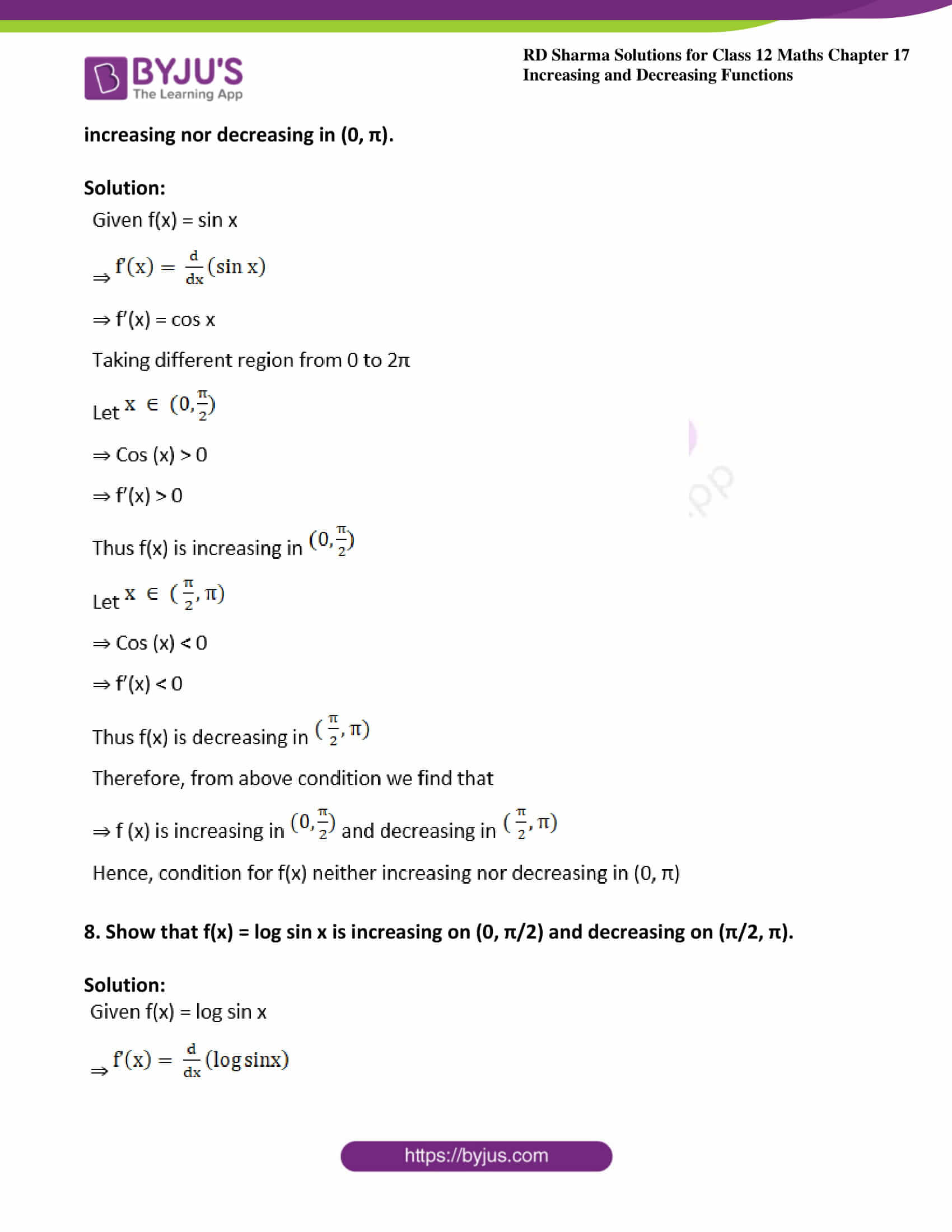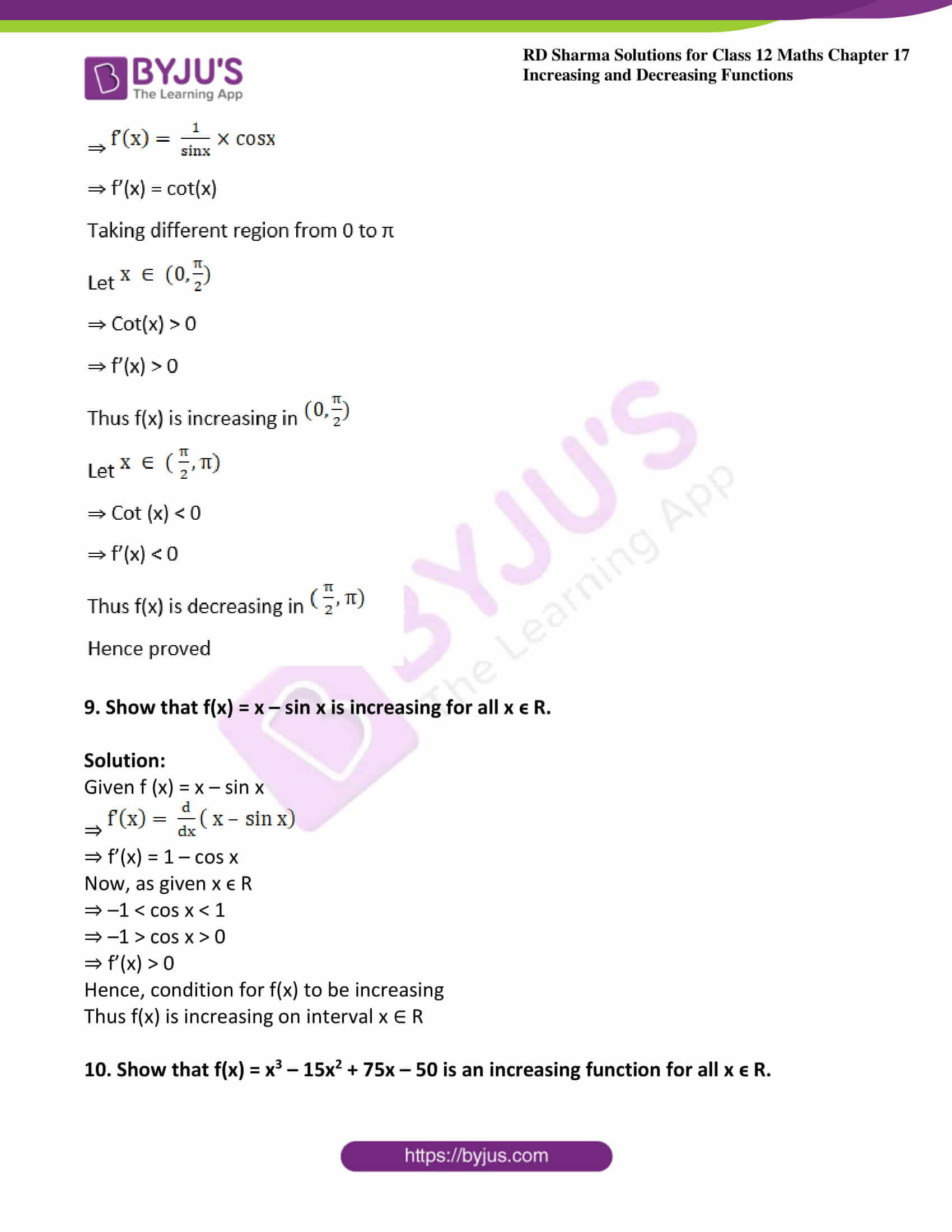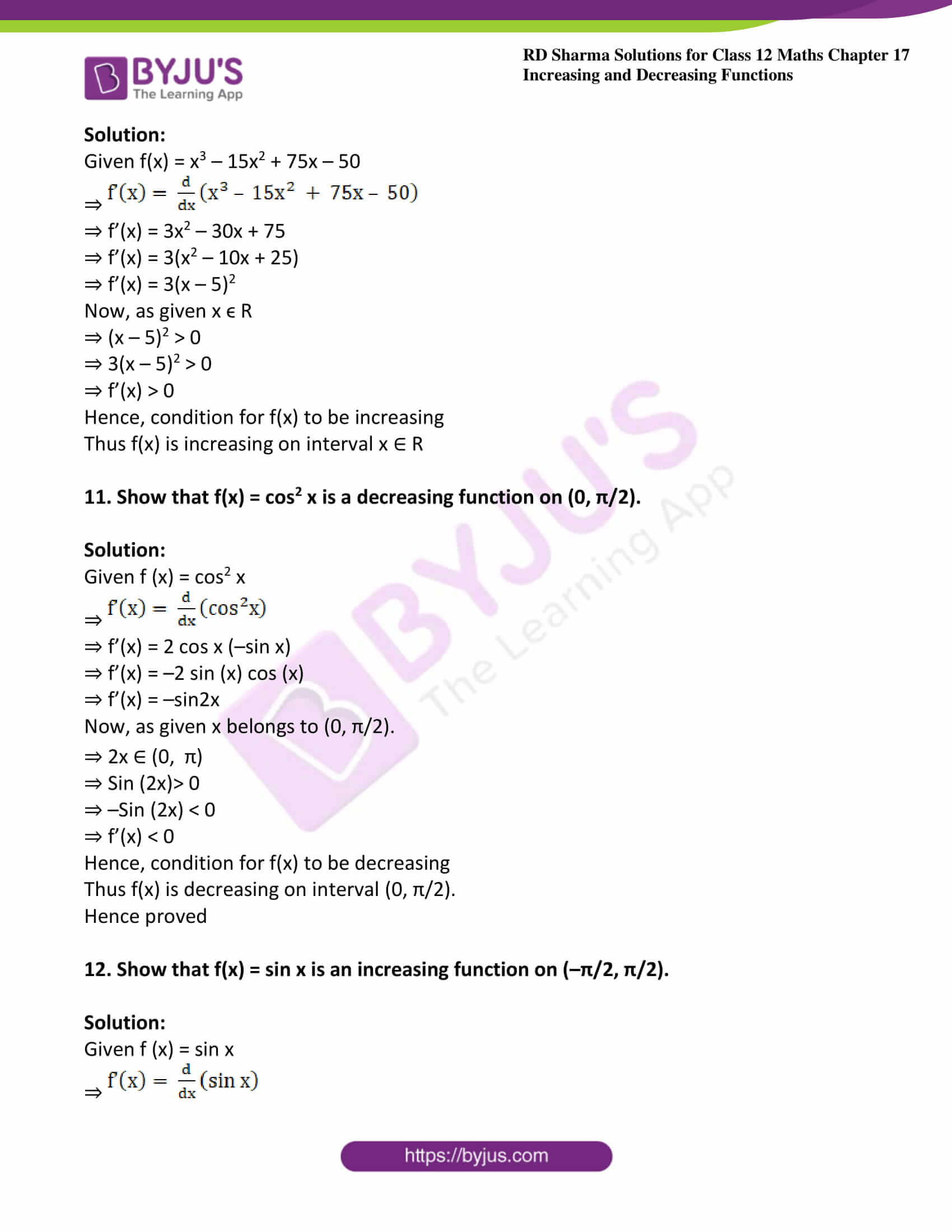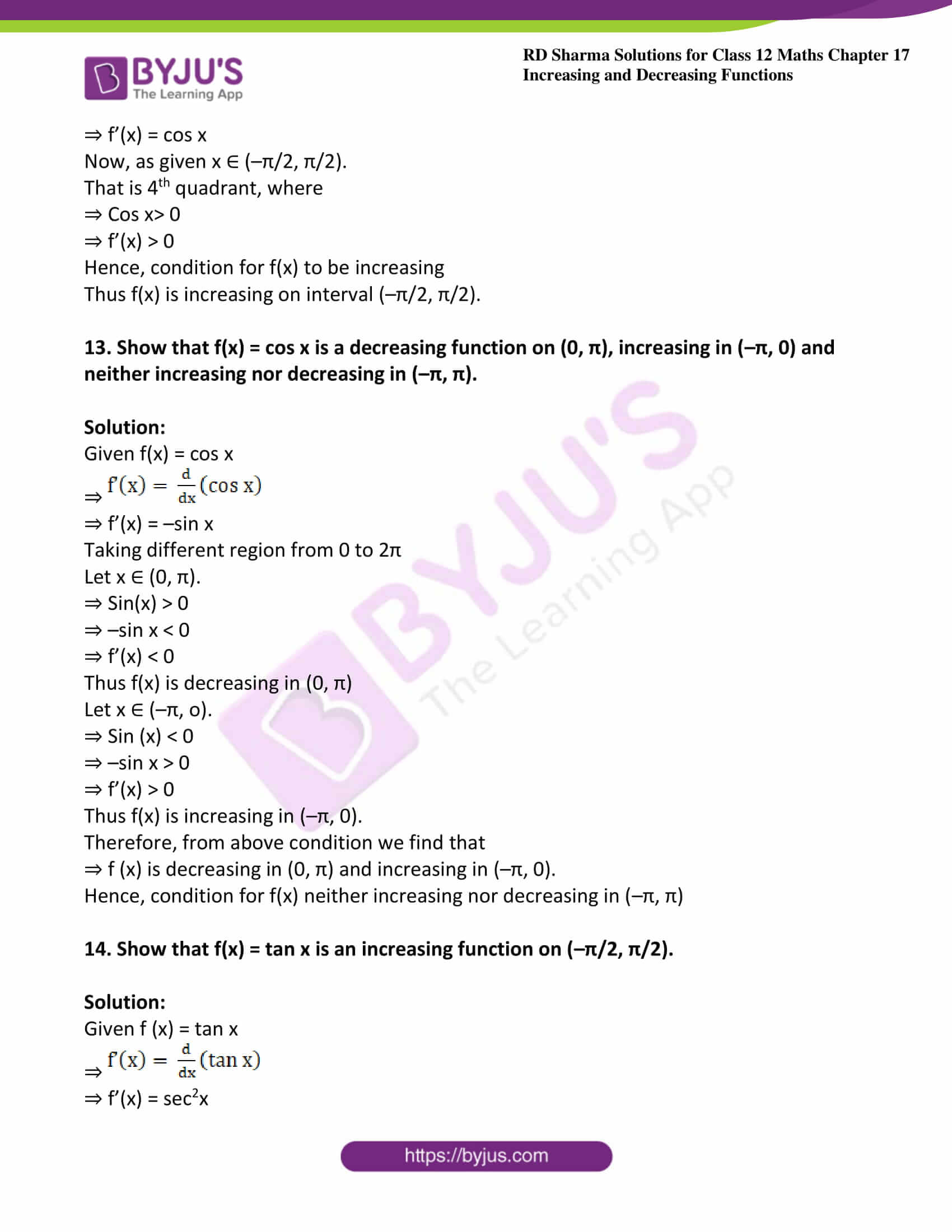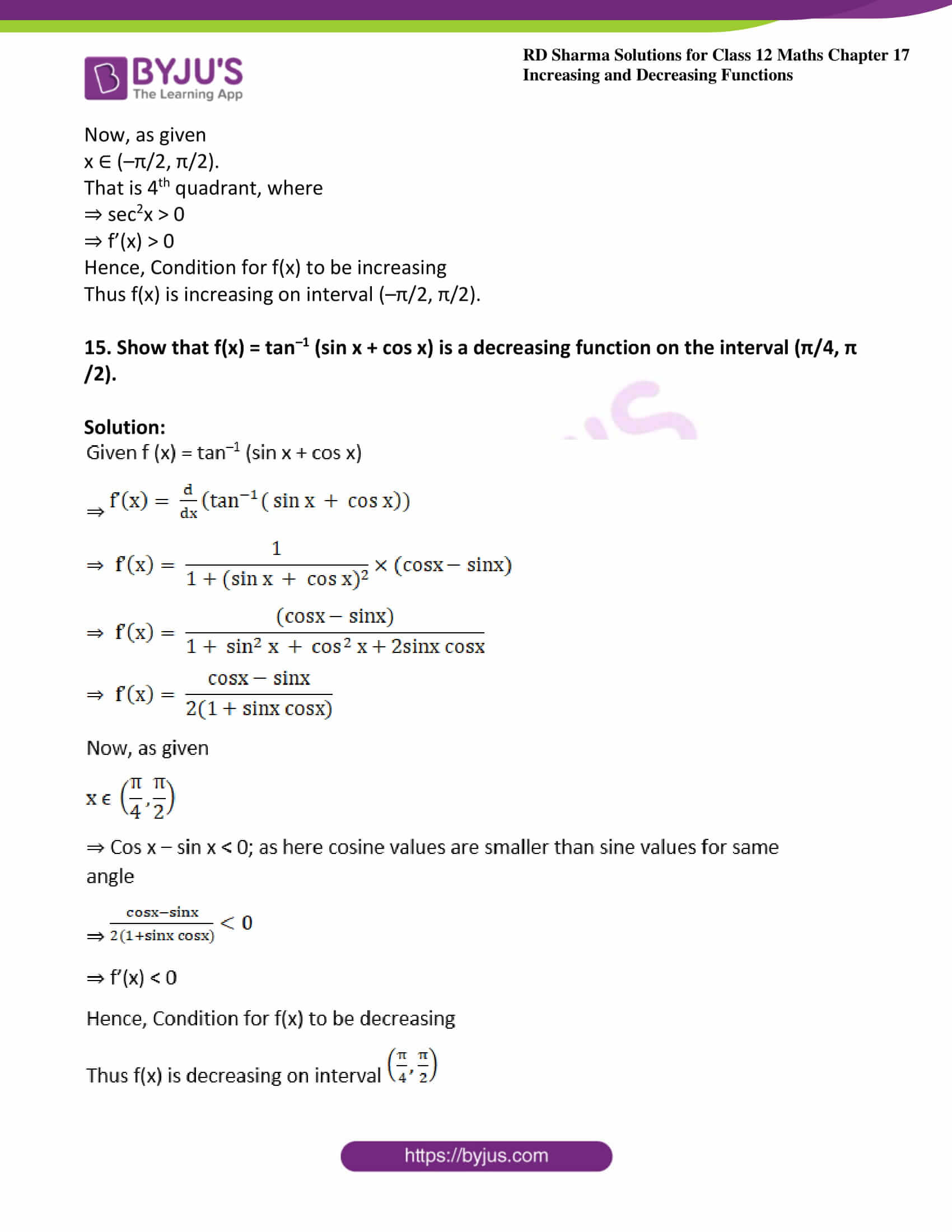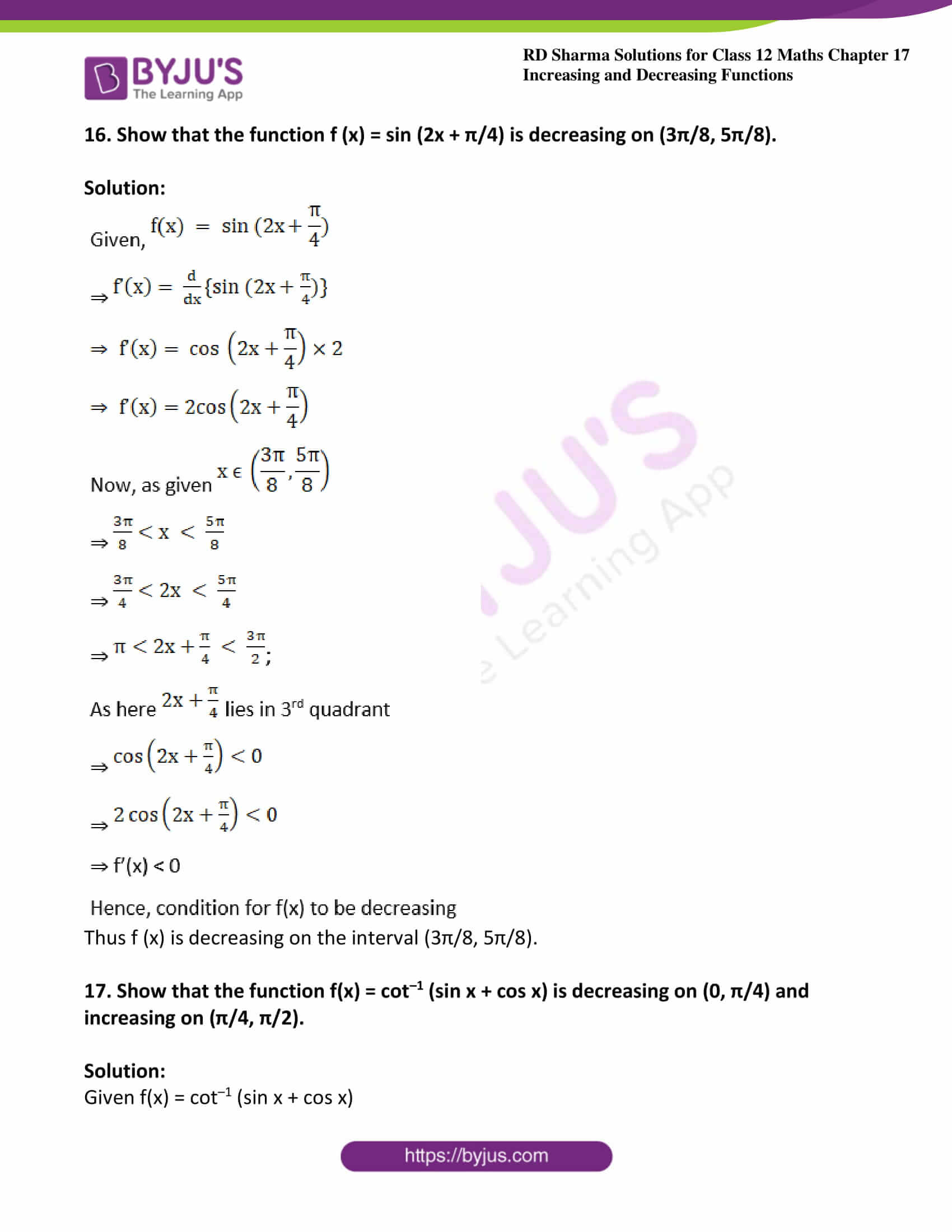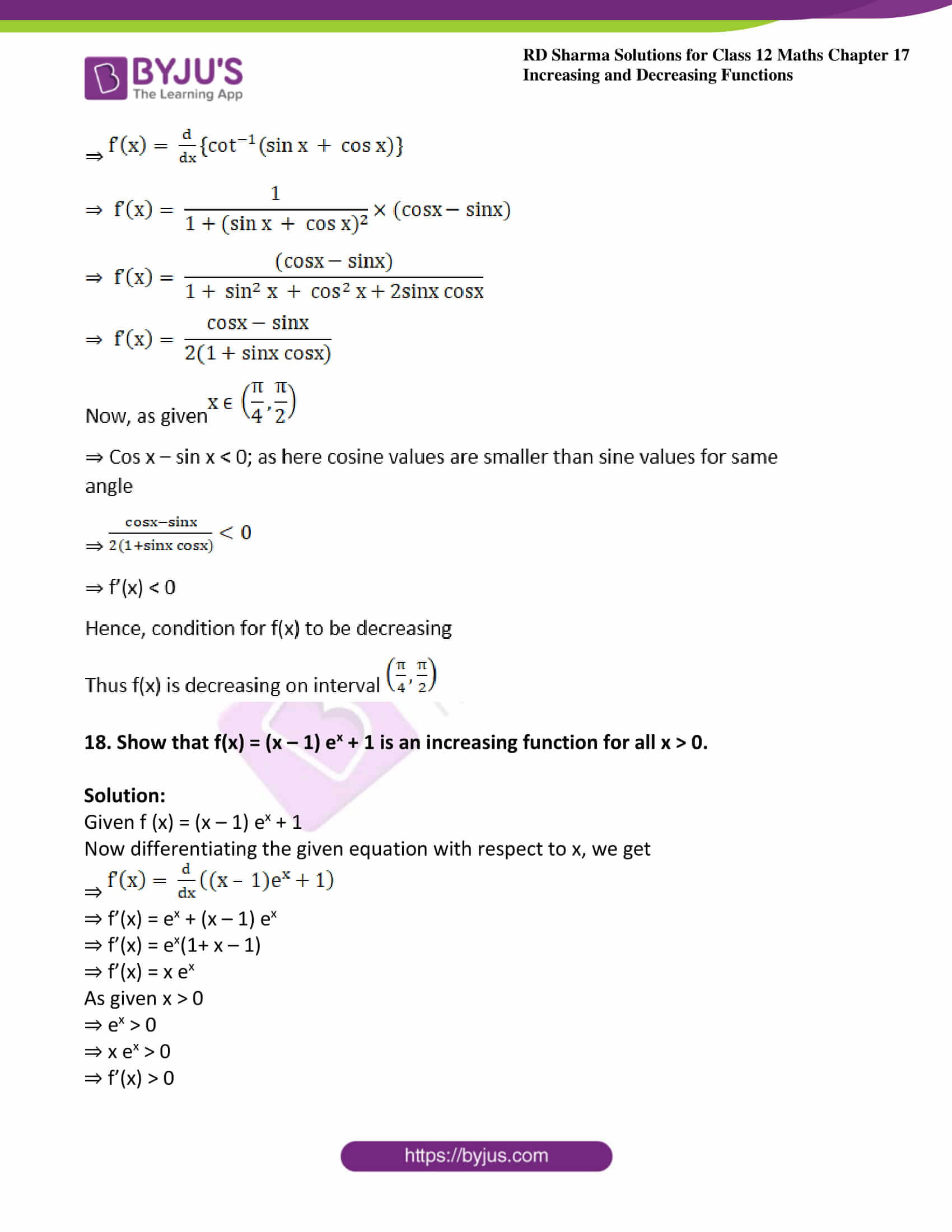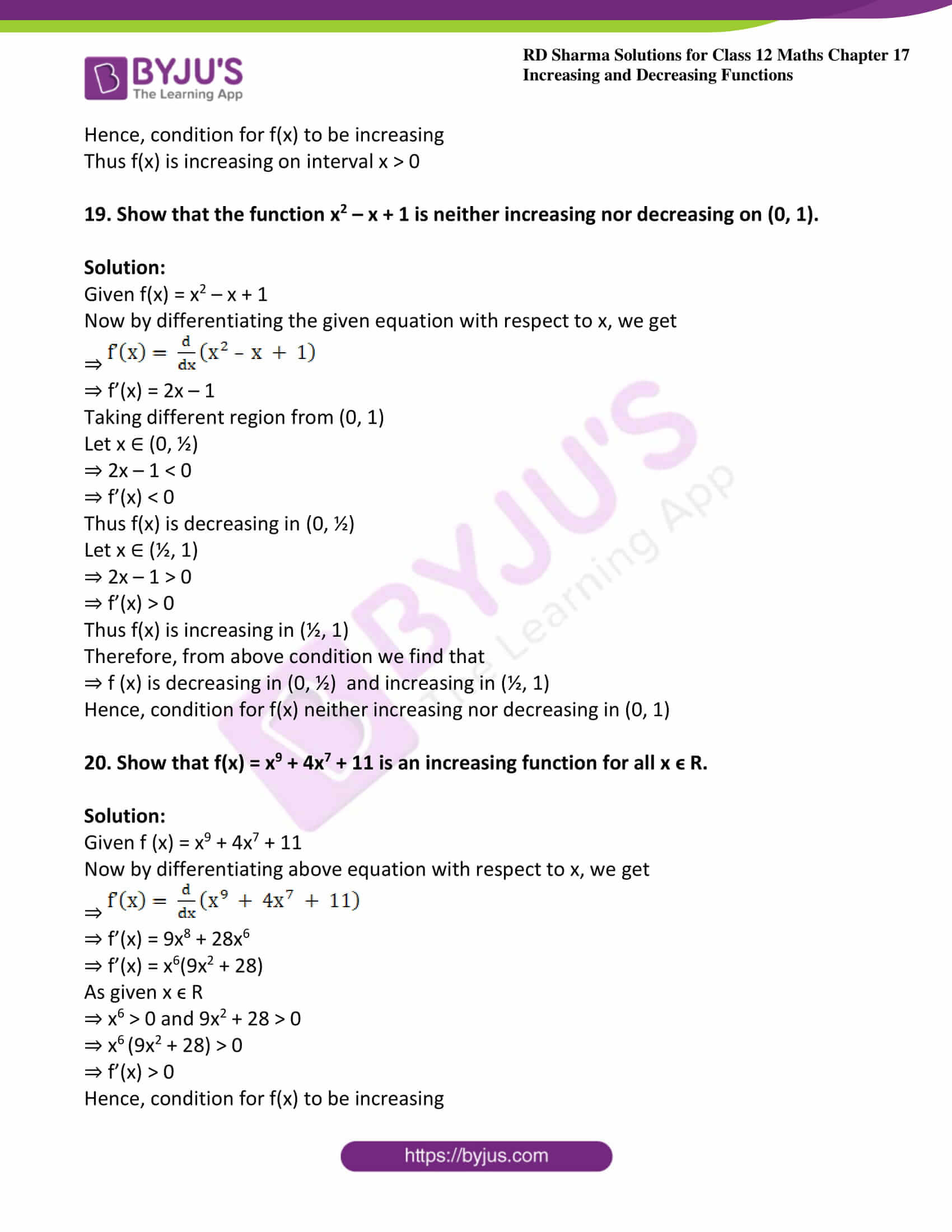### Access another exercise of RD Sharma Solutions For Class 12 Chapter 17 – Increasing and Decreasing Functions

Exercise 17.1 Solutions

### Access answers to Maths RD Sharma Solutions For Class 12 Chapter 17 – Increasing and Decreasing Functions Exercise 17.2

Exercise 17.2 Page No: 17.33

1. Find the intervals in which the following functions are increasing or decreasing.

(i) f (x) = 10 – 6x – 2x2

Solution: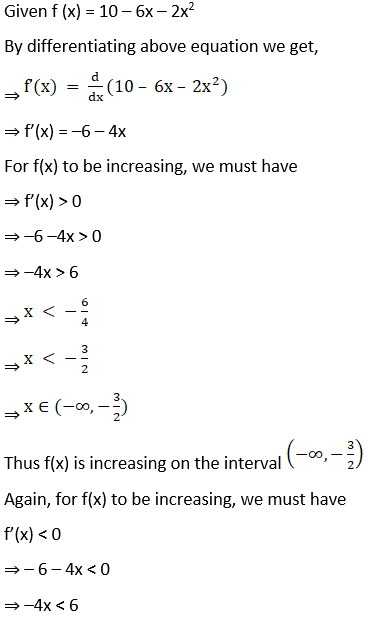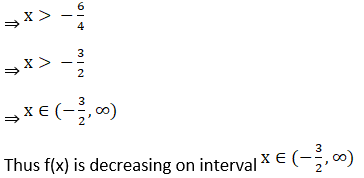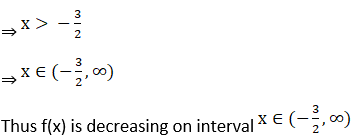(ii) f (x) = x2 + 2x – 5

Solution: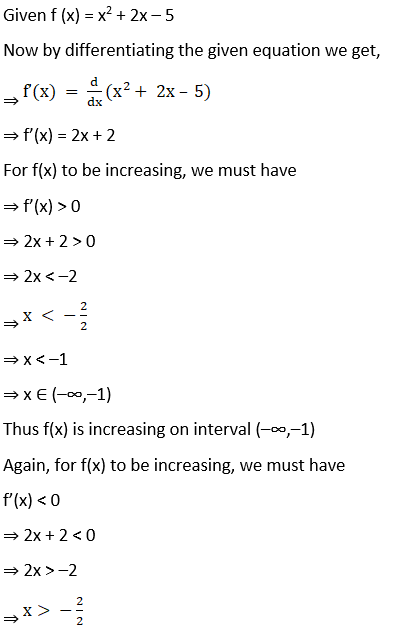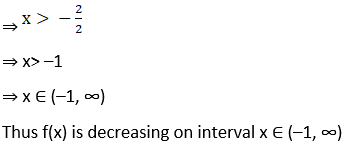(iii) f (x) = 6 – 9x – x2

Solution: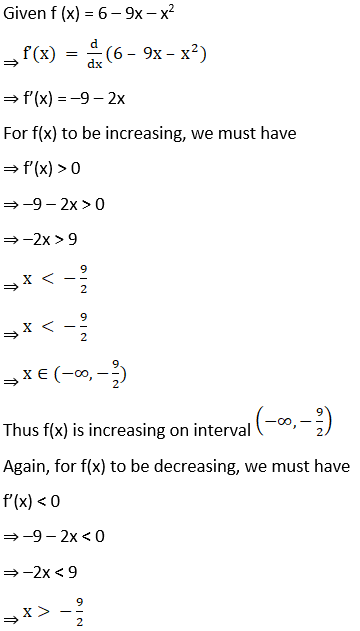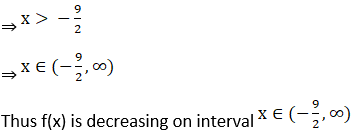(iv) f(x) = 2x3 – 12x2 + 18x + 15

Solution: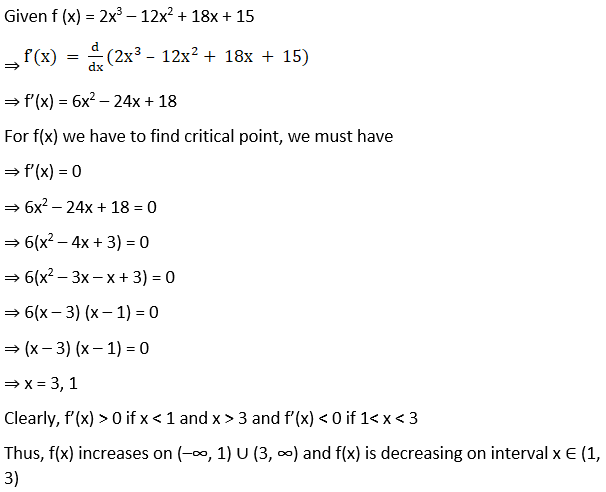(v) f (x) = 5 + 36x + 3x2 – 2x3

Solution:

Given f (x) = 5 + 36x + 3x2 – 2x3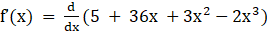⇒ f’(x) = 36 + 6x – 6x2

For f(x) now we have to find critical point, we must have

⇒ f’(x) = 0

⇒ 36 + 6x – 6x2 = 0

⇒ 6(–x2 + x + 6) = 0

⇒ 6(–x2 + 3x – 2x + 6) = 0

⇒ –x2 + 3x – 2x + 6 = 0

⇒ x2 – 3x + 2x – 6 = 0

⇒ (x – 3) (x + 2) = 0

⇒ x = 3, – 2

Clearly, f’(x) > 0 if –2< x < 3 and f’(x) < 0 if x < –2 and x > 3

Thus, f(x) increases on x ∈ (–2, 3) and f(x) is decreasing on interval (–∞, –2) ∪ (3, ∞)

(vi) f (x) = 8 + 36x + 3x2 – 2x3

Solution:

Given f (x) = 8 + 36x + 3x2 – 2x3

Now differentiating with respect to x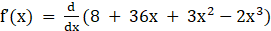⇒ f’(x) = 36 + 6x – 6x2

For f(x) we have to find critical point, we must have

⇒ f’(x) = 0

⇒ 36 + 6x – 6x2 = 0

⇒ 6(–x2 + x + 6) = 0

⇒ 6(–x2 + 3x – 2x + 6) = 0

⇒ –x2 + 3x – 2x + 6 = 0

⇒ x2 – 3x + 2x – 6 = 0

⇒ (x – 3) (x + 2) = 0

⇒ x = 3, – 2

Clearly, f’(x) > 0 if –2 < x < 3 and f’(x) < 0 if x < –2 and x > 3

Thus, f(x) increases on x ∈ (–2, 3) and f(x) is decreasing on interval (–∞, 2) ∪ (3, ∞)

(vii) f(x) = 5x3 – 15x2 – 120x + 3

Solution:

Given f(x) = 5x3 – 15x2 – 120x + 3

Now by differentiating above equation with respect x, we get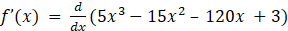⇒ f’(x) = 15x2 – 30x – 120

For f(x) we have to find critical point, we must have

⇒ f’(x) = 0

⇒ 15x2 – 30x – 120 = 0

⇒ 15(x2 – 2x – 8) = 0

⇒ 15(x2 – 4x + 2x – 8) = 0

⇒ x2 – 4x + 2x – 8 = 0

⇒ (x – 4) (x + 2) = 0

⇒ x = 4, – 2

Clearly, f’(x) > 0 if x < –2 and x > 4 and f’(x) < 0 if –2 < x < 4

Thus, f(x) increases on (–∞,–2) ∪ (4, ∞) and f(x) is decreasing on interval x ∈ (–2, 4)

(viii) f(x) = x3 – 6x2 – 36x + 2

Solution:

Given f (x) = x3 – 6x2 – 36x + 2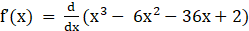⇒ f’(x) = 3x2 – 12x – 36

For f(x) we have to find critical point, we must have

⇒ f’(x) = 0

⇒ 3x2 – 12x – 36 = 0

⇒ 3(x2 – 4x – 12) = 0

⇒ 3(x2 – 6x + 2x – 12) = 0

⇒ x2 – 6x + 2x – 12 = 0

⇒ (x – 6) (x + 2) = 0

⇒ x = 6, – 2

Clearly, f’(x) > 0 if x < –2 and x > 6 and f’(x) < 0 if –2< x < 6

Thus, f(x) increases on (–∞,–2) ∪ (6, ∞) and f(x) is decreasing on interval x ∈ (–2, 6)

(ix) f(x) = 2x3 – 15x2 + 36x + 1

Solution:

Given f (x) = 2x3 – 15x2 + 36x + 1

Now by differentiating above equation with respect x, we get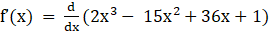⇒ f’(x) = 6x2 – 30x + 36

For f(x) we have to find critical point, we must have

⇒ f’(x) = 0

⇒ 6x2 – 30x + 36 = 0

⇒ 6 (x2 – 5x + 6) = 0

⇒ 6(x2 – 3x – 2x + 6) = 0

⇒ x2 – 3x – 2x + 6 = 0

⇒ (x – 3) (x – 2) = 0

⇒ x = 3, 2

Clearly, f’(x) > 0 if x < 2 and x > 3 and f’(x) < 0 if 2 < x < 3

Thus, f(x) increases on (–∞, 2) ∪ (3, ∞) and f(x) is decreasing on interval x ∈ (2, 3)

(x) f (x) = 2x3 + 9x2 + 12x + 20

Solution:

Given f (x) = 2x3 + 9x2 + 12x + 20

Differentiating above equation we get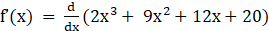⇒ f’(x) = 6x2 + 18x + 12

For f(x) we have to find critical point, we must have

⇒ f’(x) = 0

⇒ 6x2 + 18x + 12 = 0

⇒ 6(x2 + 3x + 2) = 0

⇒ 6(x2 + 2x + x + 2) = 0

⇒ x2 + 2x + x + 2 = 0

⇒ (x + 2) (x + 1) = 0

⇒ x = –1, –2

Clearly, f’(x) > 0 if –2 < x < –1 and f’(x) < 0 if x < –1 and x > –2

Thus, f(x) increases on x ∈ (–2,–1) and f(x) is decreasing on interval (–∞, –2) ∪ (–2, ∞)

2. Determine the values of x for which the function f(x) = x2 – 6x + 9 is increasing or decreasing. Also, find the coordinates of the point on the curve y = x2 – 6x + 9 where the normal is parallel to the line y = x + 5.

Solution:

Given f(x) = x2 – 6x + 9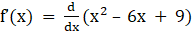⇒ f’(x) = 2x – 6

⇒ f’(x) = 2(x – 3)

For f(x) let us find critical point, we must have

⇒ f’(x) = 0

⇒ 2(x – 3) = 0

⇒ (x – 3) = 0

⇒ x = 3

Clearly, f’(x) > 0 if x > 3 and f’(x) < 0 if x < 3

Thus, f(x) increases on (3, ∞) and f(x) is decreasing on interval x ∈ (–∞, 3)

Now, let us find coordinates of point

Equation of curve is f(x) = x2 – 6x + 9

Slope of this curve is given by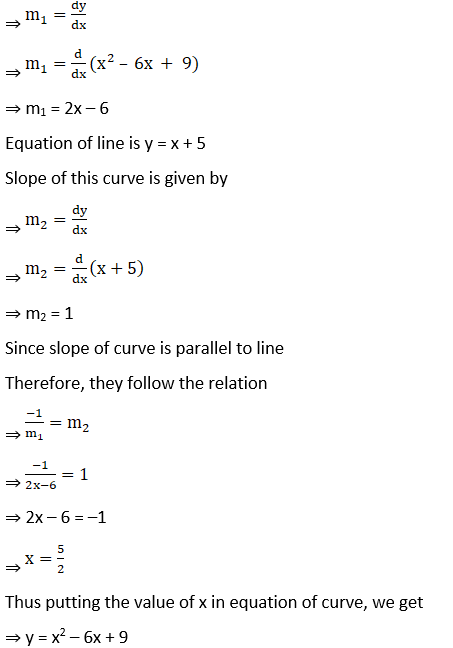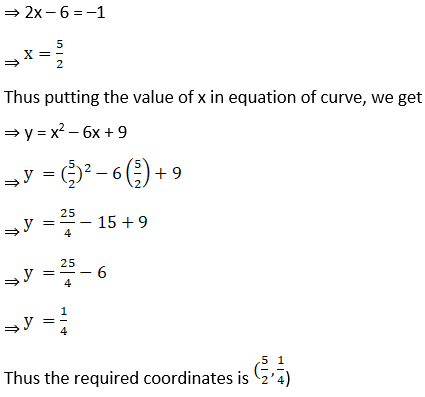3. Find the intervals in which f(x) = sin x – cos x, where 0 < x < 2π is increasing or decreasing.

Solution: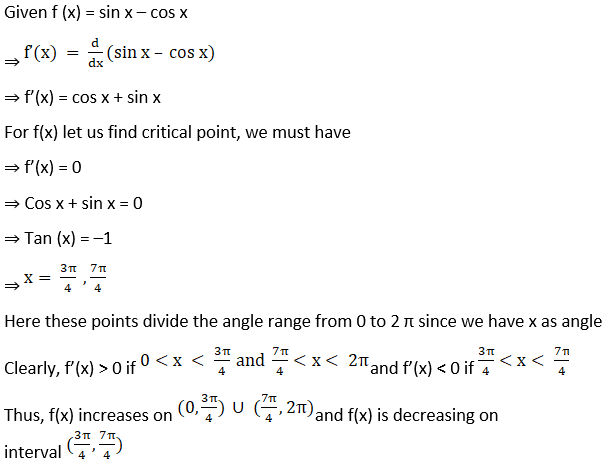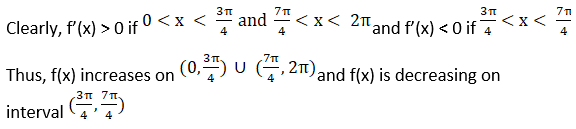4. Show that f(x) = e2x is increasing on R.

Solution:

Given f (x) = e2x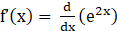⇒ f’(x) = 2e2x

For f(x) to be increasing, we must have

⇒ f’(x) > 0

⇒ 2e2x > 0

⇒ e2x > 0

Since, the value of e lies between 2 and 3

So, whatever be the power of e (that is x in domain R) will be greater than zero.

Thus f(x) is increasing on interval R

5. Show that f (x) = e1/x, x ≠ 0 is a decreasing function for all x ≠ 0.

Solution: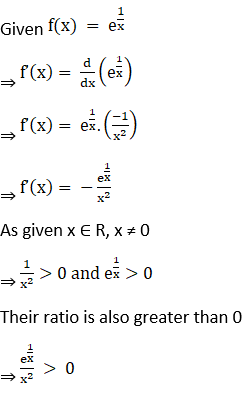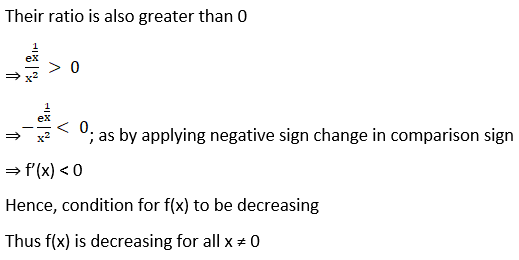6. Show that f(x) = loga x, 0 < a < 1 is a decreasing function for all x > 0.

Solution: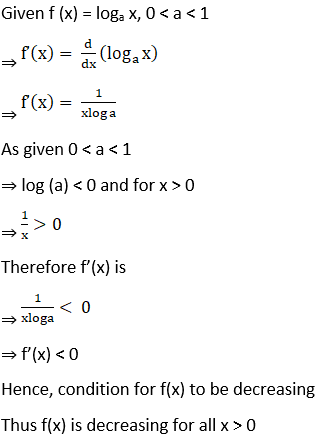7. Show that f(x) = sin x is increasing on (0, π/2) and decreasing on (π/2, π) and neither increasing nor decreasing in (0, π).

Solution: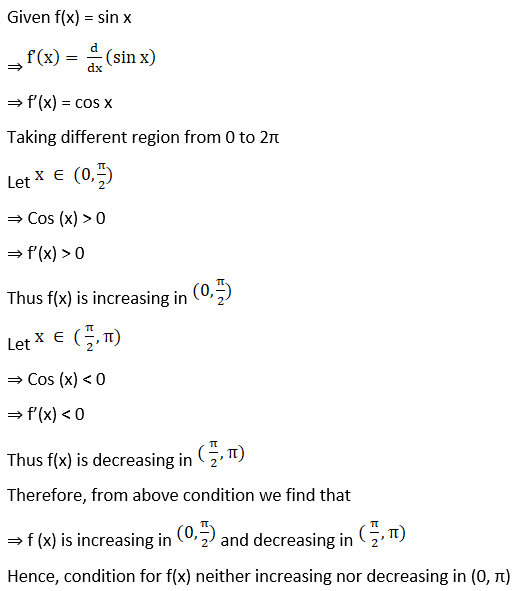8. Show that f(x) = log sin x is increasing on (0, π/2) and decreasing on (π/2, π).

Solution: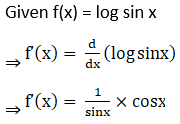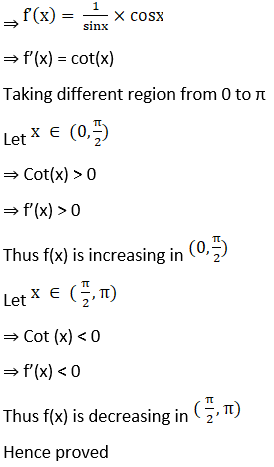9. Show that f(x) = x – sin x is increasing for all x ϵ R.

Solution:

Given f (x) = x – sin x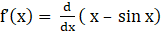⇒ f’(x) = 1 – cos x

Now, as given x ϵ R

⇒ –1 < cos x < 1

⇒ –1 > cos x > 0

⇒ f’(x) > 0

Hence, condition for f(x) to be increasing

Thus f(x) is increasing on interval x ∈ R

10. Show that f(x) = x3 – 15x2 + 75x – 50 is an increasing function for all x ϵ R.

Solution:

Given f(x) = x3 – 15x2 + 75x – 50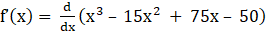⇒ f’(x) = 3x2 – 30x + 75

⇒ f’(x) = 3(x2 – 10x + 25)

⇒ f’(x) = 3(x – 5)2

Now, as given x ϵ R

⇒ (x – 5)2 > 0

⇒ 3(x – 5)2 > 0

⇒ f’(x) > 0

Hence, condition for f(x) to be increasing

Thus f(x) is increasing on interval x ∈ R

11. Show that f(x) = cos2 x is a decreasing function on (0, π/2).

Solution:

Given f (x) = cos2 x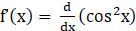⇒ f’(x) = 2 cos x (–sin x)

⇒ f’(x) = –2 sin (x) cos (x)

⇒ f’(x) = –sin2x

Now, as given x belongs to (0, π/2).

⇒ 2x ∈ (0,π)

⇒ Sin (2x)> 0

⇒ –Sin (2x) < 0

⇒ f’(x) < 0

Hence, condition for f(x) to be decreasing

Thus f(x) is decreasing on interval (0, π/2).

Hence proved

12. Show that f(x) = sin x is an increasing function on (–π/2, π/2).

Solution:

Given f (x) = sin x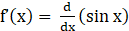⇒ f’(x) = cos x

Now, as given x ∈ (–π/2, π/2).

⇒ Cos x> 0

⇒ f’(x) > 0

Hence, condition for f(x) to be increasing

Thus f(x) is increasing on interval (–π/2, π/2).

13. Show that f(x) = cos x is a decreasing function on (0, π), increasing in (–π, 0) and neither increasing nor decreasing in (–π, π).

Solution:

Given f(x) = cos x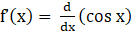⇒ f’(x) = –sin x

Taking different region from 0 to 2π

Let x ∈ (0, π).

⇒ Sin(x) > 0

⇒ –sin x < 0

⇒ f’(x) < 0

Thus f(x) is decreasing in (0, π)

Let x ∈ (–π, o).

⇒ Sin (x) < 0

⇒ –sin x > 0

⇒ f’(x) > 0

Thus f(x) is increasing in (–π, 0).

Therefore, from above condition we find that

⇒ f (x) is decreasing in (0, π) and increasing in (–π, 0).

Hence, condition for f(x) neither increasing nor decreasing in (–π, π)

14. Show that f(x) = tan x is an increasing function on (–π/2, π/2).

Solution:

Given f (x) = tan x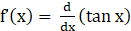⇒ f’(x) = sec2x

Now, as given

x ∈ (–π/2, π/2).

⇒ sec2x > 0

⇒ f’(x) > 0

Hence, Condition for f(x) to be increasing

Thus f(x) is increasing on interval (–π/2, π/2).

15. Show that f(x) = tan–1 (sin x + cos x) is a decreasing function on the interval (π/4, π /2).

Solution: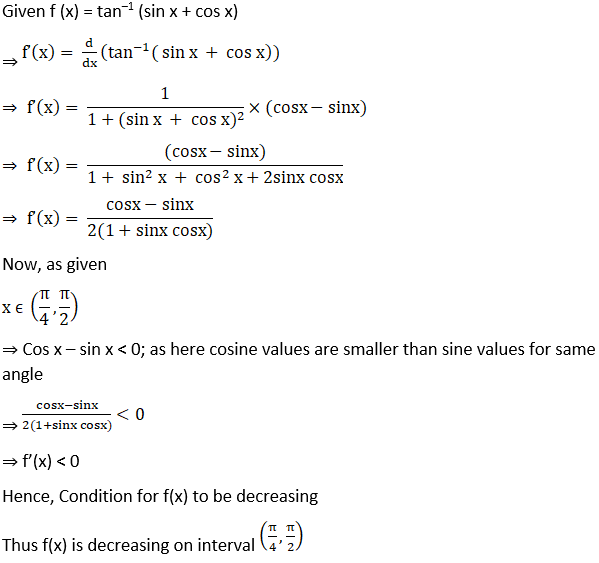16. Show that the function f (x) = sin (2x + π/4) is decreasing on (3π/8, 5π/8).

Solution: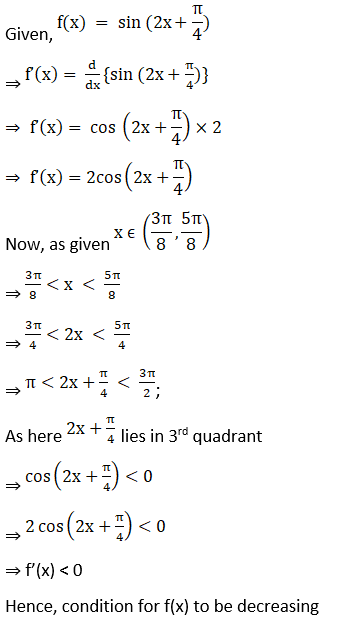Thus f (x) is decreasing on the interval (3π/8, 5π/8).

17. Show that the function f(x) = cot–1 (sin x + cos x) is decreasing on (0, π/4) and increasing on (π/4, π/2).

Solution:

Given f(x) = cot–1 (sin x + cos x)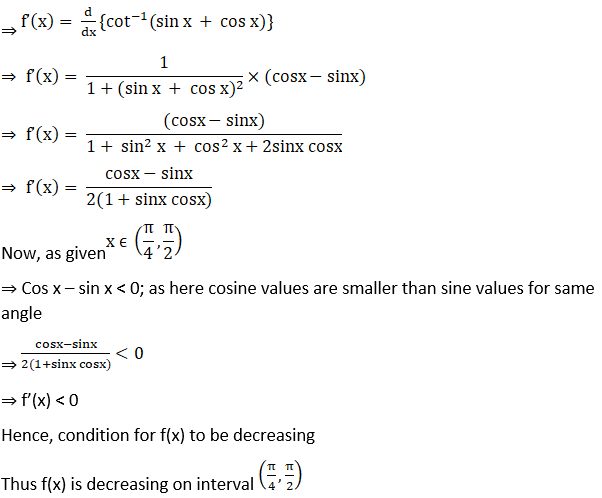18. Show that f(x) = (x – 1) ex + 1 is an increasing function for all x > 0.

Solution:

Given f (x) = (x – 1) ex + 1

Now differentiating the given equation with respect to x, we get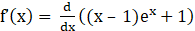⇒ f’(x) = ex + (x – 1) ex

⇒ f’(x) = ex(1+ x – 1)

⇒ f’(x) = x ex

As given x > 0

⇒ ex > 0

⇒ x ex > 0

⇒ f’(x) > 0

Hence, condition for f(x) to be increasing

Thus f(x) is increasing on interval x > 0

19. Show that the function x2 – x + 1 is neither increasing nor decreasing on (0, 1).

Solution:

Given f(x) = x2 – x + 1

Now by differentiating the given equation with respect to x, we get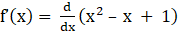⇒ f’(x) = 2x – 1

Taking different region from (0, 1)

Let x ∈ (0, ½)

⇒ 2x – 1 < 0

⇒ f’(x) < 0

Thus f(x) is decreasing in (0, ½)

Let x ∈ (½, 1)

⇒ 2x – 1 > 0

⇒ f’(x) > 0

Thus f(x) is increasing in (½, 1)

Therefore, from above condition we find that

⇒ f (x) is decreasing in (0, ½)  and increasing in (½, 1)

Hence, condition for f(x) neither increasing nor decreasing in (0, 1)

20. Show that f(x) = x9 + 4x7 + 11 is an increasing function for all x ϵ R.

Solution:

Given f (x) = x9 + 4x7 + 11

Now by differentiating above equation with respect to x, we get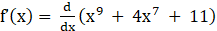⇒ f’(x) = 9x8 + 28x6

⇒ f’(x) = x6(9x2 + 28)

As given x ϵ R

⇒ x6 > 0 and 9x2 + 28 > 0

⇒ x6 (9x2 + 28) > 0

⇒ f’(x) > 0

Hence, condition for f(x) to be increasing

Thus f(x) is increasing on interval x ∈ R